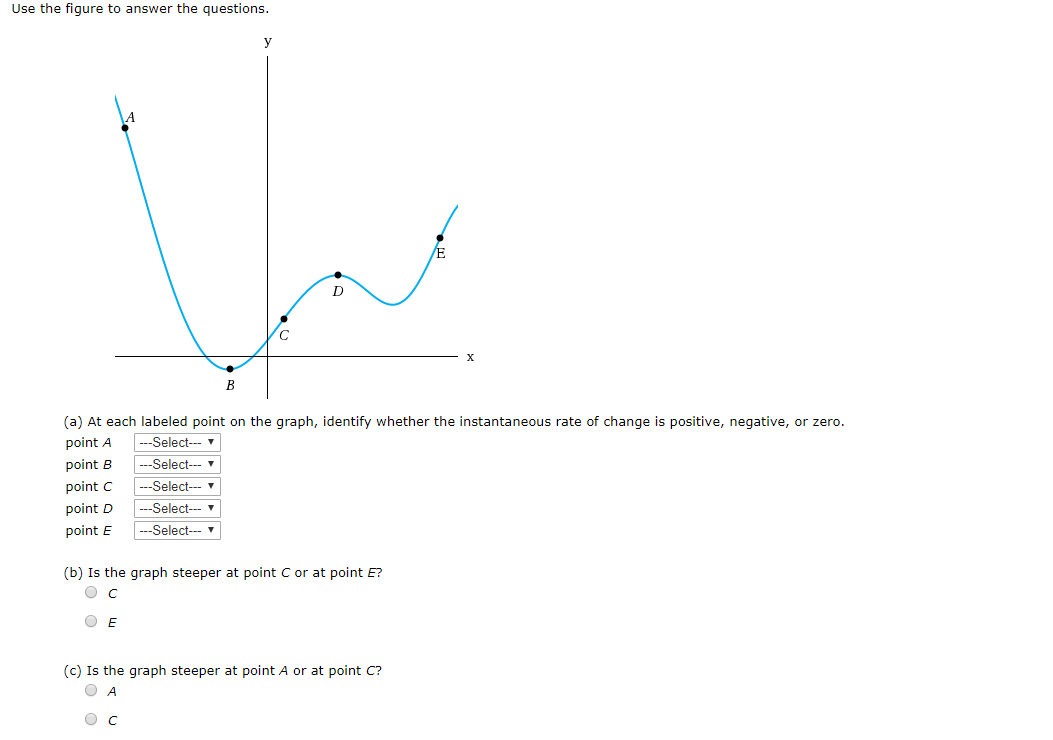# Use the figure to answer the questionsyEГВ(a) At each labeled point on the graph, identify whether the instantaneous rate of change is positive, negative, or zero.Select-Select--Selectpoint Apoint Bpoint C-Selectpoint D-Selectpoint E(b) Is the graph steeper at point C or at point E?(c) Is the graph steeper at point A or at point C?O AC

Question
3 viewshelp_outlineImage TranscriptioncloseUse the figure to answer the questions y E Г В (a) At each labeled point on the graph, identify whether the instantaneous rate of change is positive, negative, or zero. Select -Select --Select point A point B point C -Select point D -Select point E (b) Is the graph steeper at point C or at point E? (c) Is the graph steeper at point A or at point C? O A C fullscreen
check_circle

Step 1

a) At A the graph is moving downwards so there instantaneous rate of change is negative.

At B the graph has local minimum so there instantaneous rate of change is 0.

At C the graph is moving upwards so there instantaneous rate of change is positive.

At D the graph has local maximum s so there instantaneous rate of change is 0.

At E the graph is moving upwards so there instantaneous rate of change is positive.

Point B=0

Point C=positive

Point D= 0

Point E=positive

Step 2

At E the graph is increasing faster than point C. So the graph i...

### Want to see the full answer?

See Solution

#### Want to see this answer and more?

Solutions are written by subject experts who are available 24/7. Questions are typically answered within 1 hour.*

See Solution
*Response times may vary by subject and question.
Tagged in

### Calculus